# Discrepancy algorithm inspired by gradient descent and multiplicative weights; after Levy, Ramadas and Rothvoss

A week or so ago at our Theory Lunch we had the pleasure to listen to Harishchandra Ramadas (student of Thomas Rothvoss) who told us about their latest discrepancy algorithm. I think the algorithm is quite interesting as it combines ideas from gradient descent and multiplicative weights in a non-trivial (yet very simple) way. Below I reprove Spencer’sdeviations theorem with their machinery (in the actual paper Levy, Ramadas and Rothvoss do more than this).

First let me remind you the setting (see also this previous blog post for some motivation on discrepancy and a bit more context; by the way it is funny to read the comments in that post after this): givenone wants to find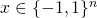(think of it as a “coloring” of the coordinates) such thatfor some numerical constant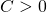(whenis a normalized vectors of‘s and‘s the quantity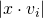represents the unbalancedness of the coloring in the set corresponding to). Clearly it suffices to give a method to findwith at least half of its coordinates equal to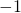andand such thatfor some numerical constant(indeed one can then simply recurse on the coordinates not yet set toor; this is the so-called “partial coloring” argument). Note also that one can drop the absolute value by takingand(the number of constraints then becomesbut this is easy to deal with and we ignore it here for sake of simplicity).

The algorithm

Let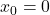,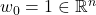. We run an iterative algorithm which keeps at every time stepa subspaceof valid update directions and then proceeds as follows. First find (using for instance a basis for)such that

(1)Then updatewhere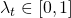is maximal so thatremains in. Finally update the exponential weights by.

It remains to describe the subspace. For this we introduce the set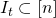containing thelargest coordinates of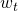(the “inactive” coordinates) and the set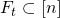containing the coordinates ofequal toor(the “frozen” coordinates). The subspaceis now described as the set of points orthogonal to (i), (ii), (iii)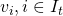, (iv). The intuition for (i) and (ii) is rather clear: for (i) one simply wants to ensure that the method keeps making progress towards the boundary of the cube (i.e.,) while for (ii) one wants to make sure that coordinates which are already “colored” (i.e., set toor) are not updated. In particular (i) and (ii) together ensures that at each step either the norm squared ofaugments by(in particular) or that one fixes forever one of the coordinates toor. In particular this means that after at mostiterations one will have a partial coloring (i.e., half of the coordinates set toor, which was our objective). Property (iii) is about ensuring that we stop walking in the directions where we are not making good progress (there are many ways to ensure this and this precise form will make sense towards the end of the analysis). Property (iv) is closely related, and while it might be only a technical condition it can also be understood as ensuring that locally one is not increasing the softmax of the constraints, indeed (iv) exactly says that one should move orthogonally to the gradient of.

The analysis

Let. Note that since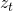is on the sphere andone has that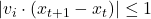. Thus using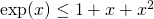for, as well as property (iv) (i.e.,) andone obtains: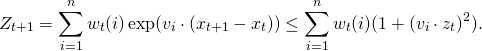Observe now that the subspacehas dimension at least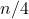(say for) and thus by (1) and the above inequalities one gets:In particular for anyfor some numerical constant. It only remains to observe that this ensuresfor any(this concludes the proof since we already observed that at time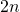at least half of the coordinates are colored). For this last implication we simply use property (iii). Indeed assume that some coordinatesatisfies at some time,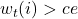for some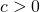. Since each update increases the weights (multiplicatively) by at mostit means that there is a previous time (say) where this weight was larger than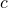and yet it got updated, meaning that it was not in the topweights, and in particular one hadwhich contradictsforlarge enough (namely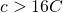).

This entry was posted in Theoretical Computer Science. Bookmark the permalink.

## One Response to "Discrepancy algorithm inspired by gradient descent and multiplicative weights; after Levy, Ramadas and Rothvoss"

•